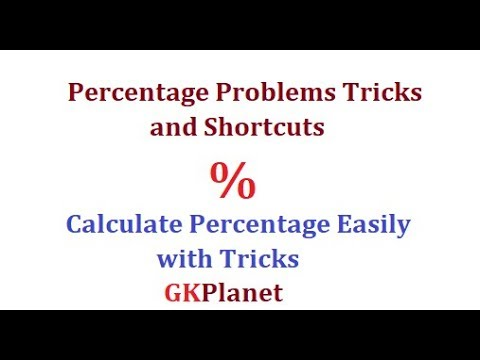# If the numerator of a fraction be increased by 15% and its denominator be diminished by 8% , the value of the fraction is 15/16. Find the original fraction.

Q. If the numerator of a fraction be increased by 15% and its denominator be diminished by 8 %, the value of the fraction is 15/16. Find the original fraction.

A. 5/4
B. 7/2
C. 8/3
D. None of these

Let the original fraction be x/y.
Then (115% of x)/(92% of y)=15/16 => (115x/92y)=15/16
((15/16)*(92/115))=3/4
Watch this video for more trick on percentageThanks for reading If the numerator of a fraction be increased by 15% and its denominator be diminished by 8% , the value of the fraction is 15/16. Find the original fraction.

←Previous
« Prev Post
Next→
Next Post »